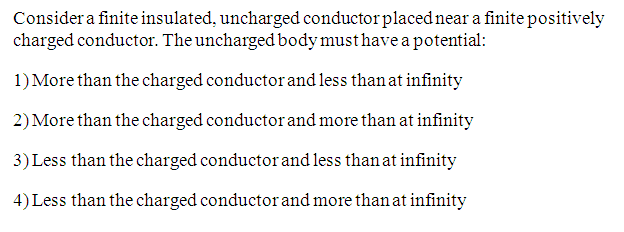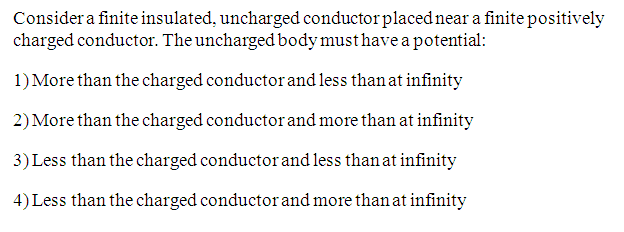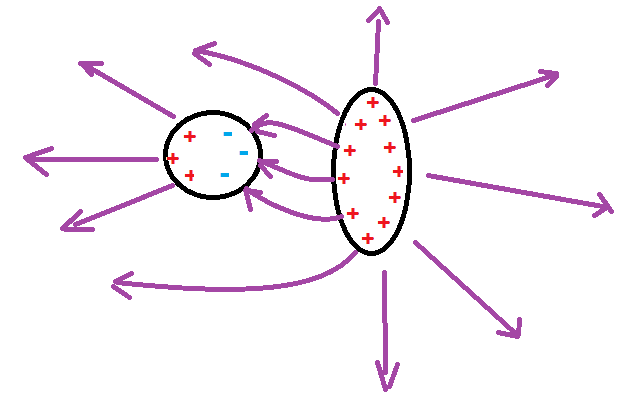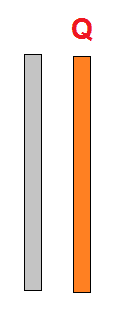# Potential of charged and uncharged conductor

Vibhor

## Homework Statement## The Attempt at a Solution

Assuming zero of the potential is at infinity , the potential refers to the potential difference between the each conductor and infinity .

The potential of the uncharged conductor would be due to three charges, positive induced charge , negative induced charge and positively charged conductor . Now contribution of the potential due to charged conductor would be less as compared to its contribution on the charged conductor itself .

But ,

Due to proximity of negative induced charges to the charged conductor , its potential ( charged conductor ) will be less as compared to the situation if uncharged conductor was not present .

So , how should I compare the potentials of the two conductors .

Thanks

Homework Helper

## Homework Statement## The Attempt at a Solution

Assuming zero of the potential is at infinity , the potential refers to the potential difference between the each conductor and infinity .

The potential of the uncharged conductor would be due to three charges, positive induced charge , negative induced charge and positively charged conductor . Now contribution of the potential due to charged conductor would be less as compared to its contribution on the charged conductor itself .

But ,

Due to proximity of negative induced charges to the charged conductor , its potential ( charged conductor ) will be less as compared to the situation if uncharged conductor was not present .

So , how should I compare the potentials of the two conductors .

Thanks
Think of the electric field lines.

•Vibhor
Vibhor
Think of the electric field lines.

Homework Helper
The potential of a conductor is the same everywhere inside and on the surface. The electric field lines emerge from charges and end in charges or in infinity. The potential drops along a field line, the gradient of potential is opposite to the direction of the field line. What do you say about the potential of the uncharged conductor with respect to the charged one? What do say about the potential of the conductors with respect to infinity?•Vibhor
Vibhor
The potential of a conductor is the same everywhere inside and on the surface. The electric field lines emerge from charges and end in charges or in infinity. The potential drops along a field line, the gradient of potential is opposite to the direction of the field line. What do you say about the potential of the uncharged conductor with respect to the charged one? What do say about the potential of the conductors with respect to infinity?
View attachment 102200
Since field lines emerge on charged conductor and ends on negative charges on uncharged one , potential of charged is higher . Again field lines emerge on both the conductors and end up at infinity .Hence , potentials of both the conductors are more than that at infinity . Option 4 ) is correct .Makes sense ?

Homework Helper
Since field lines emerge on charged conductor and ends on negative charges on uncharged one , potential of charged is higher . Again field lines emerge on both the conductors and end up at infinity .Hence , potentials of both the conductors are more than that at infinity . Option 4 ) is correct .Makes sense ?
Yes. I hope it is correct.•Vibhor
Vibhor
Ok . Would you agree that bringing an uncharged conductor near the charged one decreases the potential of charged conductor as negative induced charges will be closer .As a result their contribution to the potential at the charged one will be more than the positive induced charges ??

Homework Helper
Ok . Would you agree that bringing an uncharged conductor near the charged one decreases the potential of charged conductor as negative induced charges will be closer .As a result their contribution to the potential at the charged one will be more than the positive induced charges ??
I would not be so sure. Look at the system in the figure:These are to identical metal sheets, with lateral sizes much larger then the distance between them. One is charged, the other is neutral. What is the electric field around the charged one if it is alone? You bring the uncharged sheet in, close to the charged one. What is the electric field to the right of the charged one, between the sheets and to the left of the uncharged one?

•Vibhor
Vibhor
The electric field in every case is σ/ε0.

Homework Helper
The electric field in every case is σ/ε0.
Not quite if σ is the charge per unit area. But the electric field is the same in both cases. Assuming the potential zero somewhere far away, does it change at the charged sheet if the uncharged one is placed near to it?

Vibhor
Yes. Assuming the potential zero somewhere far away, does it change at the charged sheet if the uncharged one is placed near to it?
No . But we considered infinite plates. I think if we were to consider finite plates , the field would change .

Homework Helper
We considered finite, but large plates.
The charged object induces an uneven charge distribution on the uncharged metal. But can that charge distribution influence the surface charge on the other metal object on the farther side?
I do not know, if the potential of the charged conductor changes or not. But very far away from both pieces, the two bodies seem a system with charge Q, just like the charged body alone.
Why should be the work needed to bring in a test charge to the charged body different if there is a neutral object nearby?

Vibhor
Thanks a lot ehild. As always you have been really helpful .My thinking in this problem was unnecessarily complicated . You did a simple and nice analysis of the problem .

Last edited:
Vibhor
I am attaching an image from the textbook . Kindly have a look at it .

Thinking more about this problem I considered two identical conducting spherical shells , uncharged one A and charged one B . I believe the potential of B decreases on bringing A near B . Consider potential at the center O of B . Potential due to charges on B = ##\frac{KQ}{R}## . Potential due to induced charges on A = some negative quantity . Clearly the potential of B decreases . I might be missing something . Please help me resolve this doubt .

Thanks

#### Attachments

Homework Helper
I am attaching an image from the textbook . Kindly have a look at it .

Thinking more about this problem I considered two identical conducting spherical shells , uncharged one A and charged one B . I believe the potential of B decreases on bringing A near B . Consider potential at the center O of B . Potential due to charges on B = ##\frac{KQ}{R}## . Potential due to induced charges on A = some negative quantity . Clearly the potential of B decreases . I might be missing something . Please help me resolve this doubt .

Thanks
I did not say that the potential on the charged metal body would not change if you bring a neutral one nearby. The electric field might change, but not in all cases. I have show a situation, the parallel plates, where the electric field did not change. The original problem did not ask about the change of the potential of the charged conductor; it asked which one became greater, and if the potential of both were positive.
It is a difficult problem to calculate the potentials on conductors knowing their charges, as the charge distribution is not even in the presence of the other conductor. If you argue that after the positively charged metal sphere A made the nearby side of the neutral one B negative and the far side positive, and the charge distribution on the neutral sphere would alter the charge distribution on A in return, so the negative induced charge would induce positive charge on the near side and make the far side of A less positive, you can think further, that now the increased positive charge induce more negative charge on the near side of B, which in turn, would make the near side of A more positive, and so on, till the far side of A becomes negative which is impossible.
So I say again, I do not know, if the potential on the charged conductor decreases or not if a neutral conductor is brought nearby. Perhaps it does.
One is sure: both conductor surfaces are equipotentials, the electric field at the surfaces is perpendicular to the surface, and the surface charge density corresponds to the electric field, σ= ε0 E.

Homework Helper
Gold Member
I think you can show in general that the potential of the positively charged conductor will decrease when an uncharged conductor is brought nearby.

There's a theorem of electrostatics that states that when charges are free to move, they will arrange themselves to minimize the total electrostatic potential energy of the system. ("Thomson's theorem")

It can also be shown that the total electrostatic potential energy ##U## of a system of finite-sized conductors is $$U = \frac{1}{2}\sum_i Q_i V_i$$ where ##Q_i## and ##V_i## are the net charge and potential of the ##i##th conductor and the sum is over all conductors.

Apply this formula before and after the uncharged conductor is brought nearby.

•Vibhor
Vibhor
Thank you very much TSny . Thanks ehild .